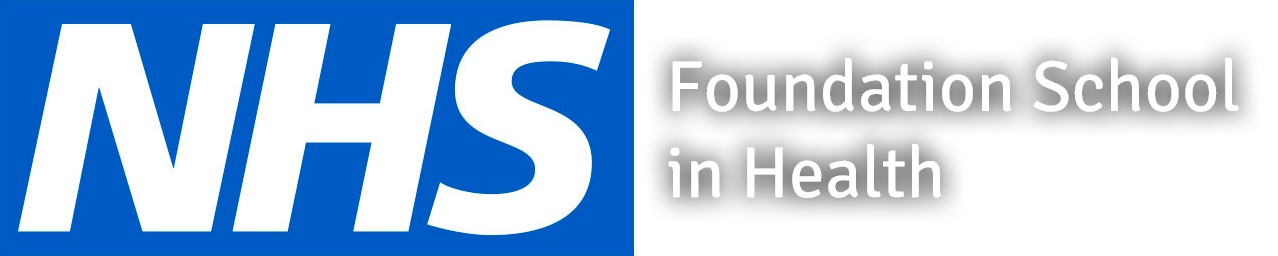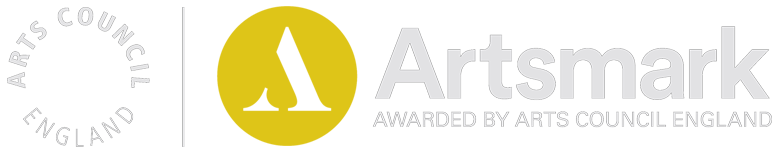Mathematics A Level

Mathematics is a high currency A Level subject which is valued by employers and further education establishments alike. The course is an exciting journey where students will develop their mathematical knowledge and start to see how the different strands of mathematics interlink and thread together. It introduces concepts and ideas that will challenge all students but also provides a set of core skills that will complement many other A Level courses. Further Mathematics is offered as an additional challenge only to be faced by those students who are the most able mathematicians and relish the thought of studying mathematics for at least half of their timetable.

## Exam Board

A Level Mathematics and Further Mathematics are both Edexcel courses.

## Course Content

The A Level Mathematics course is a linear, two year course that consists of Pure Mathematics and Applied Mathematics (Mechanics & Statistics). Pure Mathematics makes up two thirds of the course and consists of such topics as: algebra; trigonometry; vectors; proof; calculus; exponentials and logarithms. Applied Mathematics makes up the remaining third of the course. The Mechanics aspect is closely related to Physics and consists of such topics as the study of forces, acceleration and moments. Statistics supports many Social Sciences and gives a good grounding in statistical techniques often used in research and data analysis. Topics studied include probability distributions and hypothesis testing. For A Level Further Mathematics, the Pure Mathematics content of the A Level course is extended to include concepts containing more abstract ideas such as: complex number theory; polar co-ordinates; understanding of mathematical proof; hyperbolic functions and differential equations. In addition, the study of Decision Mathematics is also introduced. Course assessments and requirements The A Level Mathematics course is continually assessed throughout Year 12 and Year 13 with a series of assessment points. Students must pass each assessment point to show competency for that unit of work and display the necessary skills for what is to come later in the course. A mock exam is then taken at the end of Year 12.   All A Level Mathematics students will need to have the Casio FX-991EX Classwiz calculator. The A Level course is formally assessed by 100% examination at the end of Year 13. This consists of 3 two hour papers. These are 2 Pure papers and 1 Applied paper (assessing both Mechanics and Statistics). For students doing the A Level Further Mathematics course, they will study the full A Level course in Year 12 with their formal exams (outlined above), taken at the end of Year 12. This is followed by the Further Mathematics course in Year 13.  The students will then sit 4 formal examinations in their chosen modules at the end of Year 13.

## Beyond the Classroom

As well as attending Mathematics lessons students will need to dedicate some time each week to external study, re-enforcing the vitally essential basics learned in lessons and honing their skills. A "Brew and Review" support session is provided after school one night each week in the Mathematics Department. In this session students can work on their subject skills with each other and get support from their teachers whilst having a hot drink.